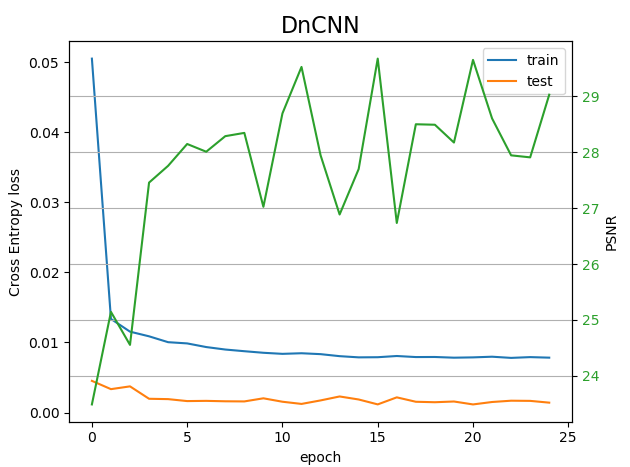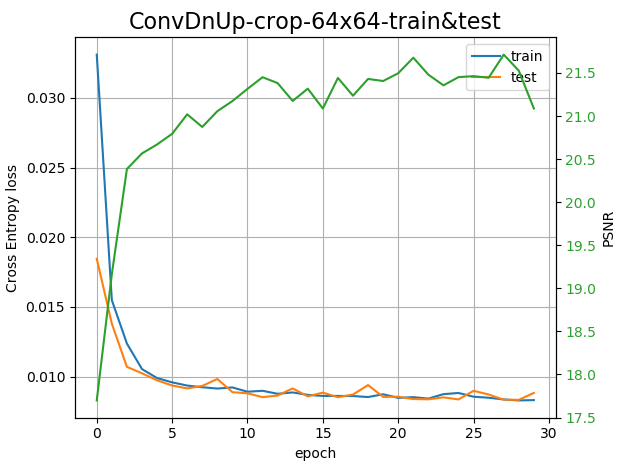# Denoiser models : loss value depends on cropping size?

I face a problem that puzzling me. I intend to use several denoiser architectures to evaluate their performances for my use-case. In the following I show the train/test losses as well as the Peak Signal to Noise Ratio (PSNR) which a kind of accuracy metric between an input image (original) and the denoised version if one adds a noise to the original. So, schematically

``````I_orig -> "add noise" -> I_noisy=I_orig + Noise ->  "denoise" -> I_denoised
``````

and then compute the MSEloss(I_orig, I_denoised) and the PSNR.

So, far so good. Now, let me give some details. Concerning the training phase

``````def train(args, model, device, train_loader, transforms, optimizer, epoch, use_clipping=True)
model.train()

transf_size = len(transforms)

train_loss = 0 # to get the mean loss over the dataset

batch_size = len(img_batch)
new_img_batch = torch.zeros(batch_size,1,args.crop_size,args.crop_size)

for i in range(batch_size):
# perform data augmentation to get new_img_batch
(... same code ...)

new_img_batch_noisy = new_img_batch \
+ args.sigma_noise*torch.randn(*new_img_batch.shape)

if use_clipping:
scaler = MinMaxScaler()
for i in range(batch_size):
new_img_batch[i] = scaler(new_img_batch[i])
new_img_batch_noisy[i] = scaler(new_img_batch_noisy[i])

# send the inputs and target to the device
new_img_batch,  new_img_batch_noisy = new_img_batch.to(device), \
new_img_batch_noisy.to(device)

# perform the optimizer loop
outputs = model(new_img_batch_noisy)
loss = F.mse_loss(outputs, new_img_batch)
train_loss += loss.item()
loss.backward()
optimizer.step()

``````

For the test this is essentially the same code but

1. I switch to `model.eval()`
2. I use `with torch.no_grad():`

Well, here are some results, using either a homemade Convolutional network or the DnCNN model which uses Conv2d and BatchNorm layers from https://github.com/cszn/DnCNN/blob/master/TrainingCodes/dncnn_pytorch/main_train.pyWhat is puzzling me is why the test loss is so low compared to train loss ??? The two sets are originating to the same larger set of 512x512 images. I use random cropping 64x64 and rotation/flip data augmentation for the train set, in contrast for the test I do not use data augmentation (ie. neither random cropping and flip/rot). The batch_size is 50, 100 for the train and test sets respectively, and the size of the two sets are 5000 and 1000 respectively.

Any idea is welcome.

Ha! I give a new information: the loss depends on the cropping size, if I choose 64x64 for the test as it is for the train, thenThe test loss = train loss (nb. the PSNR is a bit lower as I have adapted a little the definition so the max-min range is defined with the pixel values not the dtype values)

Is there a way to get the loss independent of the size of the image?

I have cross-checked that both train & test losses have the same dependence wrt the cropping size:

``````loss( 64x64 cropping) ~ (3-4) * loss (256x256 cropping)
``````

They do not change (very little) if I modify (x2, /2) the batch size, the number of images in the datasets.

I have x-checked that the `F.mse_loss` applied to Nx1xHxW tensors is such that the mean takes as normalization factor NxHxW: I have done a brute-force 3for-loops computation.

Now, it is generally states that learning on 64x64 patches would in general miss some details of larger images (ie 512x512).
But

1. this statement is only valid if the pixel resolution is increased between the two sets of images. Here, my 64x64 patches have rigorously the same resolution than the 512x512 ones, as the patches are originated from random cropping of 512x512 pixels images.
2. I would imagine the 512x512 loss (ie. the test loss) be worse that the 64x64 loss, which is not the case.

Any idea?